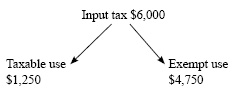# GST rules for financial service providers - deductions from output tax

2005 amendment to the GST rules for financial service providers prevents taxpayers from claiming deductions of input tax twice.

section 20C of the Goods and Services Tax Act 1985

### Introduction

A change has been made to the application of section 20C of the Goods and Services Tax Act 1985 to ensure that the section reflects its policy intent. The change modifies the application of item "a" contained in the formula in section 20C and prevents taxpayers from being able to claim deductions of input tax twice.

### Background

Sections 20(3)(h) and 20C were inserted into the GST Act by the Taxation (GST, Trans-Tasman Imputation and Miscellaneous Provisions) Act 2003 as part of a number of amendments to implement the zero-rating of business-to-business supplies of financial services. Section 20(3)(h) allows taxpayers a deduction of input tax for exempt supplies of financial services made to another financial services provider. The deduction is calculated according to a formula in section 20C.

The formula contained in section 20C has been modified by changing the definition of item "a".

The value of the deduction calculated under section 20C was previously as follows:

 a x b c x d e

Where:

1. is the total amount that the registered person would be able to deduct under section 20(3), other than under section 20(3)(h), in respect of the taxable period if all supplies of financial services were taxable supplies:
2. is the total value of exempt supplies of financial services by the registered person to the direct supplier in respect of the taxable period:
3. is the total value of supplies by the registered person in respect of the taxable period:
4. is the total value of taxable supplies by the direct supplier in respect of the taxable period, determined under section 20C:
5. is the total value of supplies by the direct supplier in respect of the taxable period, determined under section 20C.

The formula provides a proportional deduction of input tax and is in addition to that which can be recovered as a deduction of input tax if the taxpayer's principal purpose is that of making taxable supplies or by way of a change-in-use adjustment.

The proportion is found by multiplying two fractions. The first fraction is the proportion of the total value of supplies made by a taxpayer that consists of exempt supplies of financial services to a recipient financial services provider (the direct supplier). The second fraction is the proportion of the total value of supplies made by the direct supplier that consists of taxable supplies (including zero-rated supplies of financial services). The result of these fractions is multiplied by the amount in respect of which input tax could be claimed if all supplies by the taxpayer were taxable supplies.

The formula is limited to the activities of the direct supplier. Further supplies of financial services - for example, by the direct suppliers to a third or subsequent financial services provider - are not included in the formula.

A problem arose because the formula assumed that when calculating the value of item "a", the taxpayer was unable to deduct input tax at all. This is not always the case. If a taxpayer had been able for any reason to deduct GST paid on the purchase of goods and services the application of "a" in the formula could have allowed taxpayers to deduct input tax twice, once in respect of the initial deduction and again under the formula.

The change was introduced during the Finance and Expenditure Committee's consideration of the bill.

### Key features

Item "a" of the formula in section 20C has been modified to ensure that when a taxpayer has deducted input tax under section 20(3) a further deduction is not allowed under the formula.

Item "a" is now defined by:

1. isolating the total value of input tax that cannot be claimed under section 20(3) of the GST Act; and
2. allowing a deduction of the input tax calculated in (i).

The value of the deduction allowed under section 20(3)(h) is found by completing the rest of the formula.

### Application date

The amendment applies from 1 January 2005.

 Example Finance Co is completing its monthly GST return. It has recorded expenditure of \$54,000 including GST of \$6,000. Finance Co attributes the input tax of \$6,000 between its taxable and exempt supplies as follows:For the purposes of calculating item "a" in the formula Finance Co uses the input tax identified for exempt use (\$4,750) rather than the total \$6,000.